# Fractions + natural numbers - math problems

#### Number of problems found: 34

• Reminder and quotientThere are given the number C = 281, D = 201. Find the highest natural number S so that the C:S, D:S are with the remainder of 1,
• Unknown numberIf I reduced the sum of the numbers 70 and the unknown number three times, I would get 100. what is the unknown number?
• FractionFraction ? write as fraction a/b, a, b is integers numerator/denominator.
• Drawing from a hatWhen drawing numbers from a hat from 1 to 35, we select random given numbers. What is the probability that the drawn numbers will be divisible by 8 and 2?
• Balls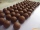From the bag with numbered balls (numbers 1,2,3,. ..20) we pick one ball. What is the probability of choosing a number containing 1?
• Ratio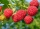Alena collected 7.8 kg of blueberries, 2.6 kg of blackberries, and 3.9 kg of cranberries. Express the ratio in the smallest natural numbers in this order.
• Milk cratesA farmer sells milk in crates that hold 15 bottles. She has 34,125 bottles of milk. How many crates can the farmer fill?
• Steps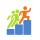Peter walked a distance of 60 m 102 steps. How many steps must he take to walk 150 m?
• Prime divisorsFind 2/3 of the ratio of the sum and the product of all prime divisors of the number 120.Divide number 135 into two additions so that one adds 30 more than 2/5 of the other add. Write the bigger one.
• NumbersDetermine the number of all positive integers less than 4183444 if each is divisible by 29, 7, 17. What is its sum?
• Homework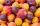In the crate are 18 plums, 27 apricot and 36 nuts. How many pieces of fruit left in the crate when Peter took 8 ninth: 1. nuts 2. apricots 3. fruit 4. drupe
• LibraryNew books were purchased for the library. Five-eighths were professional books, one-fifth were encyclopedias, and 231 books were dictionaries. How many professional books were there?
• Candies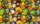There are 150 candies in the bowl. Ivan took 7 thirtieths, Charles 6 fifteenths, and Thomas 3 tenths. How many sweets are left in the bowl?
• Three machines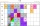The power of the three machines is 2: 3: 5. Two most powerful machines produce 400 parts per hour. How many components make all three machines in 3 hours?
• Proportion 3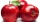For every 8 mango trees in the orchard, there are 4-star apple trees. If there are 1320 trees, how many trees of each kind are there?
• Camp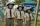In the camp are children. 1/2 went on a trip, 1/4 went to bathe and 38 children remained in the room. How many children are in camp?
• Ornamental shrubsChildren committed to plant 240 ornamental shrubs. Their commitment however exceeded by 48 shrubs. Write ratio of actually planted shrubs and commitment by lowest possible integers a/b.
• Reminder and quotientThere are given numbers A = 135, B = 315. Find the smallest natural number R greater than 1 so that the proportions R:A, R:B are with the remainder 1.
• The number 72The number 72 increase by 25%. By how much % will you have to reduce the number you created to get the number 72 again?

Do you have an interesting mathematical word problem that you can't solve it? Submit a math problem, and we can try to solve it.

We will send a solution to your e-mail address. Solved examples are also published here. Please enter the e-mail correctly and check whether you don't have a full mailbox.

Please do not submit problems from current active competitions such as Mathematical Olympiad, correspondence seminars etc...

Need help calculate sum, simplify or multiply fractions? Try our fraction calculator.